Courses

# Solutions of Reflection of Light (Page No- 200) - Physics By Lakhmir Singh, Class 10 Class 10 Notes | EduRev

## Class 10 : Solutions of Reflection of Light (Page No- 200) - Physics By Lakhmir Singh, Class 10 Class 10 Notes | EduRev

The document Solutions of Reflection of Light (Page No- 200) - Physics By Lakhmir Singh, Class 10 Class 10 Notes | EduRev is a part of the Class 10 Course Lakhmir Singh & Manjit Kaur Solutions: Class 10 Science.
All you need of Class 10 at this link: Class 10

Page No:200

Question 42: Between which two points of concave mirror should an object be placed to obtain a magnification of:
(a) -3
(b) +2.5
(c) – 0.4

Solution : (a) Between focus and centre of curvature.
(b) Between pole and focus.
(c) Beyond the centre of curvature

Question 43: At what distance from a concave mirror of focal length 10 cm should an object be placed so that:
(a) its real image is formed 20 cm from the mirror ?
(b) its virtual image is formed 20 cm from the mirror ?

Solution :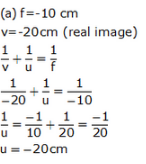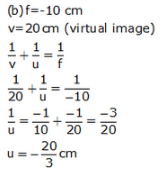Question 44: If a concave mirror has a focal length of 10 cm, find the two positions where an object can be placed to give, in each case, an image twice the height of the object.

Solution : f = -10cm

Case 1: m = 2 (Image is virtual and erect)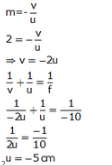Case 2:m = -2 (Image is real and inverted)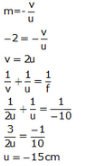Question 45: A mirror forms an image which is 30 cm from an object and twice its height.
(a) Where must the mirror be situated ?
(b) What is the radius of curvature ?
(c) Is the mirror convex or concave ?

Solution : Let the image formed is virtual and erect,
v - u = 30cm
m = 2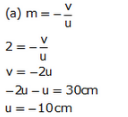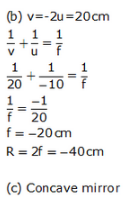Page No:205

Question 1: What type of image/images are formed by :
(a) a convex mirror ?
(b) a concave mirror ?

Solution : (a) Virtual and erect
(b) Virtual and erect; real and inverted

Question 2: Which mirror has a wider field of view ?

Solution : Convex mirror has a wider field of view.

Question 3: If you want to see an enlarged image of your face, state whether you will use a concave mirror or a convex mirror ?

Solution : Concave mirror.

Question 4: Which mirror always produces a virtual, erect and diminished image of an object ?

Solution : Convex mirror always produces a virtual, erect and diminished image.

Question 5: An object is placed at a long distance in front of a convex mirror of radius of curvature 30 cm. State the position of its image.

Solution : Image is formed at the focus.
focus = 30/2 = 15 cm
i.e. 15 cm behind the convex mirror.

Question 6: Name the spherical mirror which can produce a real and diminished image of an object.

Solution : Concave mirror.

Question 7: Name the spherical mirror which can produce a virtual and diminished image of an object.

Solution : Convex mirror.

Question 8: One wants to see a magnified image of an object in a mirror. What type of mirror should one use ?

Solution : Concave mirror should be used to get a magnified image.

Question 9: Name the mirror which can give :
(a) an erect and enlarged image of an object.
(b) an erect and diminished image of an object.

Solution : (a) Concave mirror.
(b) Convex mirror.

Question 10: State whether the following statement is true or false :

A diverging mirror is used as a rear-view mirror.

Solution : True.

Question 11: What type of mirror could be used :
(a) as a shaving mirror ?
(b) as a shop security mirror ?

Solution : (a) Concave mirror.
(b) Convex mirror.

Question 12: Which type of mirror is usually used as a rear-view mirror in motor cars ?

Solution : Convex mirror.

Question 13: What kind of mirrors are used in big shopping centres to watch the activities of the customers ?

Solution :Convex mirrors.

Question 14: A ray of light going towards the focus of a convex mirror becomes parallel to the principal axis after reflection from the mirror. Draw a labelled diagram to represent this situation.

Solution : diagram 47.

Question 15: Fill in the following blank with a suitable word :

A ray of light which is parallel to the principal axis of a convex mirror, appears to be coming from…….. after
reflection from the mirror.

Solution : focus.

Question 16: Why does a driver prefer to use a convex mirror as a rear-view mirror in a vehicle ?

Solution : A driver prefers to use a convex mirror as a rear-view mirror because
(i) A convex mirror always produces an erect image of the objects.
(ii) A convex mirror has wider field of view.

Question 17: Why can you not use a concave mirror as a rear-view mirror in vehicles ?

Solution : We cannot use a concave mirror as a rear-view mirror in vehicles because a concave mirror produces inverted images of distant objects. So, all the vehicles will be seen running upside down in the mirror.

Question 18: Where would the image be formed by a convex mirror if the object is placed :
(a) between infinity and pole of the mirror ?
(b) at infinity ?
Draw labelled ray-diagrams to show the formation of image in both the cases.

Solution : (a) Image will form between pole and focus.
(b) At focus.

Page No:206

Question 19: The shiny outer surface of a hollow sphere of aluminium of radius 50 cm is to be used as a mirror :
(a) What will be the focal length of this mirror ?
(b) Which type of spherical mirror will it provide ?
(c) State whether this spherical mirror will diverge or converge light rays.

Solution : (a) R = 50cm
f = ?
We know that
f = R/2 = 50/2 = 25 cm
(b) Convex mirror
(c) It will diverge light rays.

Question 20: What is the advantage of using a convex mirror as a rear-view mirror in vehicles as compared to a plane mirror ? Illustrate your answer with the help of labelled diagrams.

Solution : The advantage of using a convex mirror as a rear-view mirror in vehicles as compared to a plane mirror is that a convex mirror has a wider field of view as compared to a plane mirror. This enables driver to view much larger area of the traffic behind him.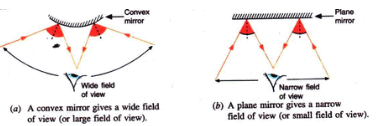Question 21: Give two uses of a convex mirror. Explain why you would choose convex mirror for these uses.

Solution : Two uses of convex mirror:
(i) A convex mirror is used as a rear-view mirror in vehicles because it forms erect and dimished images of the objects and has a wider field of view.
(ii) Big convex mirrors are used as security mirror in shops so that a large number of goods displayed in the shop can be seen in the convex mirror.

Question 22: What would your image look like if you stood dose to a large :
(a) convex mirror ?
(b) concave mirror ?

Solution : (a) Our image will be diminished, virtual and erect. This is because when the object lies anywhere between the pole and inifinity, the concave mirror forms a diminished, virtual and erect image.
(b) Our image will be enlarged, virtual and erect. This is because when the object lies within the focus of a concave mirror, it forms an enlarged, virtual and erect image.

Question 23: Which of the following are concave mirrors and which convex mirrors ?
Shaving mirrors, Car headlight mirror, Searchlight mirror, Driving mirror, Dentist’s inspection mirror, Torch mirror, Staircase mirror in a double-decker bus, Make-up mirror, Solar furnace mirror, Satellite TV dish, Shop security mirror.

Solution : Shaving mirror – concave mirror.
Car headlight mirror – concave mirror.
Searchlight mirror – concave mirror.
Driving mirror – convex mirror.
Dentist’s inspection mirror – concave mirror.
Torch mirror – concave mirror.
Staircase mirror in a double-decker bus – convex mirror.
Make-up mirror – concave mirror.
Solar furnace mirror – concave mirror.
Satellite TV dish – concave mirror.
Shop security mirror – convex mirror.

Question 24: How will you distinguish between a plane mirror, a concave mirror and a convex mirror without touching them ?

Solution : We can distinguish between a plane mirror, a concave mirror and a convex mirror by bringing our face close to each mirror, turn by turn. If the image is of the same size as our face, it is a plane mirror. If the image is magnified, it is a concave mirror. If the image is diminished, it is a convex mirror.

Question 25: If a driver has one convex and one plane rear-view mirror, how would the images in each mirror appear different ?

Solution : The images formed in the convex rear-view mirror will be smaller than those formed in the plane rear-view mirror.

Question 26: (a) Draw a labelled ray diagram to show the formation of image of an object by a convex mirror. Mark
clearly the pole, focus and centre of curvature on the diagram.
(b) What happens to the image when the object is moved away from the mirror gradually ?
(c) State three characteristics of the image formed by a convex mirror.

Solution : (a) Virtual and erect.
(b) Virtual and erect; real and inverted.

Page No:207

Question 37: The diagrams show the appearance of a fork when placed in front of and close to two mirrors A and B, turn by turn.
(a) Which mirror is convex?
(b) Which mirror is concave?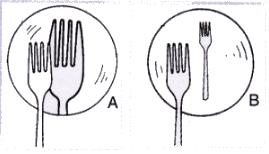Solution : (a) Mirror B is convex since it forms a smaller image of fork.
(b) Mirror A is concave since it forms a larger image of fork.

Question 38: The diagram shows a dish antenna which is used to receive television signals from a satellite. The antenna (signal detector) is fixed in front of the curved dish.
(a) What is the purpose of the dish?
(b) Should it be concave or convex?
(c) Where should the antenna be positioned to receive the strongest possible signals?
(d) Explain what change you would expect in the signals if a larger dish was used.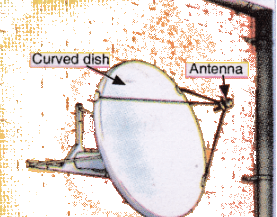Solution : (a) The purpose of the dish is to collect a large amount of TV signals from the satellite.
(b) Concave.
(c) At the focus of the dish.
(d) Stronger signals will be received.

Question 39: A man standing in front of a special mirror finds his image having a very small head, a fat body and legs of normal size. What is the shape of :
(a) top part of the mirror ?
(b) middle part of the mirror ?
(c) bottom part of the mirror ?

Solution : (a) Convex – Because a convex mirror forms a diminished image when the object is placed near it.
(b) Concave – Because a concave mirror forms an enlarged image when the object is placed near it.
(c) Plane – Because a plane mirror forms an image of same size as the object.

Question 40: Two big mirrors A and B are fitted side by side on a wall. A man is standing at such a distance from the wall that he can see the erect image of his face in both the mirrors. When the man starts walking towards the mirrors, he finds that the size of his face in mirror A goes on increasing but that in mirror B remains the same
(a) mirror A is concave and mirror B is convex
(b) mirror A is plane and mirror B is concave
(c) mirror A is concave and mirror B is convex
(d) mirror A is convex and mirror B is plane

Solution : (c) Mirror A is concave and mirror B is concave

Page No:209

Question 1: An object is kept at a distance of 5 cm in front of a convex mirror of focal length 10 cm. Calculate the position and magnification of the image and state its nature.

Solution :

u = -5cm f -10cm

We know that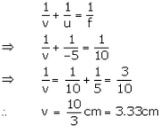The position of the image is 3.33 cm behind the convex mirror. Magnification. m= -v/u = -3.33/-5 = 0.66 The image is virtual and erect.

Question 2: An object is placed at a distance of 10 cm from a convex mirror of focal length 5 cm.
Draw a ray-diagram showing the formation of image.
State two characteristics of the image formed.
Calculate the distance of the image from mirror.

Solution :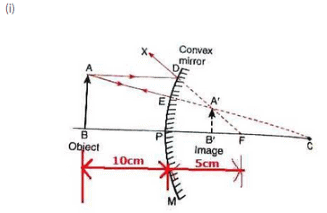(ii) The image formed is diminished and erect.
(iii) u=-10cm. f = 5cm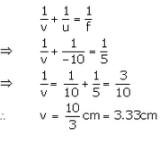Question 3: An object is placed at a distance of 6 cm from a convex mirror of focal length 12 cm. Find the position and nature of the image.

Solution : u= -6cm f = 12cm. v = ?

We know that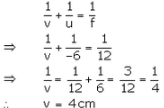Image will be formed 4 cm behind the mirror.

Since the image is formed behind the convex mirror, it is virtual and erect.

Question 4: An object placed 20 cm in front of a mirror is found to have an image 15 cm (a) in front of it, (b) behind the mirror. Find the focal length of the mirror and the kind of mirror in each case.

Solution : (a) u =-20cm, v = -15cm

We know that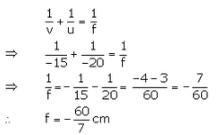The mirror is a concave mirror.
(b) u = -20cm v = 15cm

We know that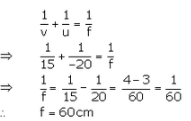The mirror is a convex mirror.

Question 5: An arrow 2.5 cm high is placed at a distance of 25 cm from a diverging mirror of focal length 20 cm. Find the nature, position and size of the image formed.

Solution : h= 2.5cm.u = -25cm. f = 20cm

We know that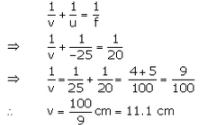The image is formed 11.1cm behind the convex mirror.

Now.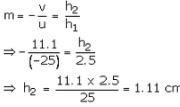The image is virtual. erect and 1.11cm tall.

Question 6: A convex mirror used as a rear-view mirror in a car has a radius of curvature of 3 m. If a bus is located at a distance of 5 m from this mirror, find the position of image. What is the nature of the image ?

Solution : Ra = 3 m  u = -5m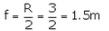We knoe that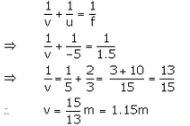The image is formed 1.15 m behind the mirror.

The image is virtual and erect.

Question 7: A diverging mirror of radius of curvature 40 cm forms an image which is half the height of the object. Find the object and image positions.

Solution : R = 40cm

f = R/2 = 10/2 = 20tm

Image is half the height of the object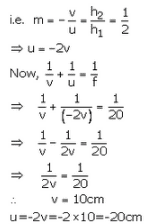So. the object is placed 20 cm in front of the mirror and the image is formed 10cm behind the mirror.

Question 8: The radius of curvature of a convex mirror used as a rear view mirror in a moving car is 2.0 m. A truck is coming from behind it at a distance of 3.5 m. Calculate (a) position, and (b) size, of the image relative to the size of the truck. What will be the nature of the image ?

Solution :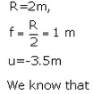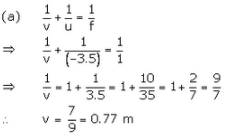So, the image is formed 0.77m behind the mirror.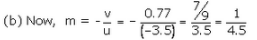As m is positive, so image formed is virtual and erect

Question 9: (a) Draw a diagram to represent a convex mirror. On this diagram mark principal axis, principal focus F
and the centre of curvature C if the focal length of convex mirror is 3 cm.
(b) An object 1 cm tall is placed 30 cm in front of a convex mirror of focal length 20 cm. Find the size and position of the image formed by the convex mirror.

Solution :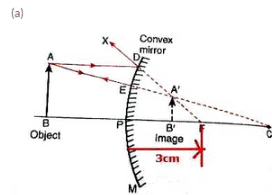(b) h1 = 1cm. u = -30cm f = 20cm. h2= ?, v = ?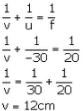The image is formed 12 cm behind the mirror.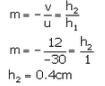Question 10: A shop security mirror 5.0 m from certain items displayed in the shop produces one-tenth magnification.
(a) What is the type of mirror ?
(b) What is the radius of curvature of the mirror ?

Solution :

(a) The mirror is of convex type.
(b) u = -5cm. m = 1/10

We have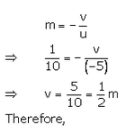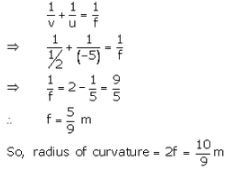Page No:210

Question 11: An object is placed 15 cm from (a) a converging mirror, and (b) a diverging mirror, of radius of curvature 20 cm. Calculate the image position and magnification in each case.

Solution :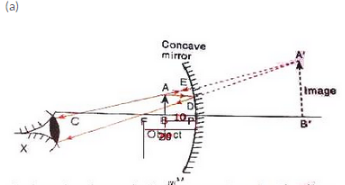fig. formation of image by the 'concave mirror when the object Is palced between its pole and focus.

(b) f = -20cm. u = -10cm. v= ?

We know that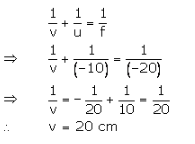(c) Characteristics of image formed
(i) Image is virtual.
(ii) Image is erect.

Question 12: An object 20 cm from a spherical mirror gives rise to a virtual image 15 cm behind the mirror. Determine the magnification of the image and the type of mirror used.

Solution : u = -20cm v = 15cm (virtual image)

We know that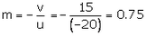The mirror used is of convex type.

Offer running on EduRev: Apply code STAYHOME200 to get INR 200 off on our premium plan EduRev Infinity!

61 docs

,

,

,

,

,

,

,

,

,

,

,

,

,

,

,

,

,

,

,

,

,

,

,

,

;